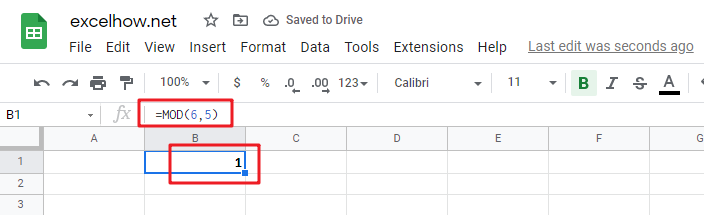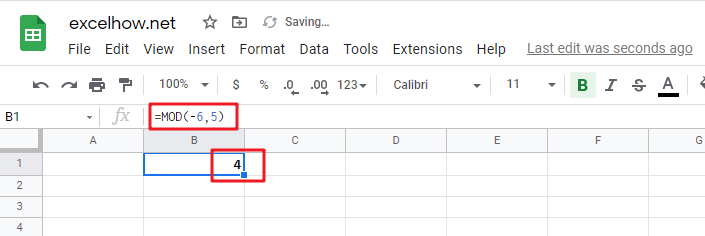# ExcelHow

This post will guide you how to use Google Sheets MOD function with syntax and examples in Google Spreadsheets.

## Description

The Google Sheets MOD function returns the remainder of two numbers after division. So you can use the MOD function to get the remainder after a number is divided by a divisor in Google Sheets. And the returned result will have the same sign as divisor.

The MOD function is a build-in function in Google Sheets and it is categorized as a Math and Trigonometry Function.

## Syntax

The syntax of the MOD function is as below:

`=MOD (number, divisor)`

Where the MOD function arguments are:

• `number` – This is a required argument.The number that you want to get the remainder.
• `divisor` – This is a required argument. The number that you want to divide number.

Note:

• If the divisor is 0, then the MOD function will return the #DIV/0! Error.
• The MOD function returns a result in the same sign as the divisor.

## Google Sheets MOD Function Examples

The below examples will show you how to use Google Sheets MOD Function to get the remainder after number is divided by divisor.

1# to get the remainder of 6/5, enter the following formula in Cell B1.

`=MOD(6,5)`2# 1# to get the remainder of -6/5, enter the following formula in Cell B1.

`=MOD(-6,5)`